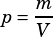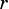The density,, of an object can be calculated using the formula, whereis the mass of the object andis its volume. The volume of a sphere can be calculated using the formula, whereis the radius of the sphere. Which of the following equations could be used to find the radius of a spherical object given its mass and density?
A)
B)
C)
D)